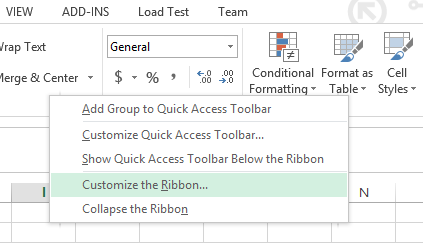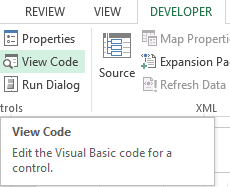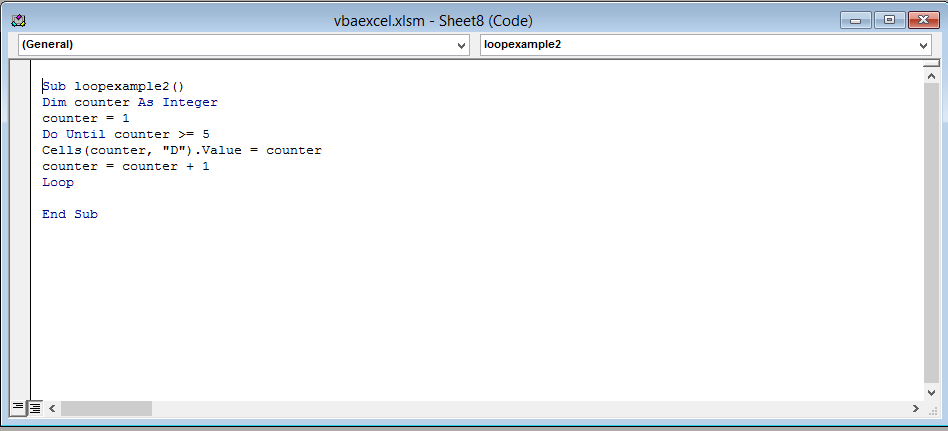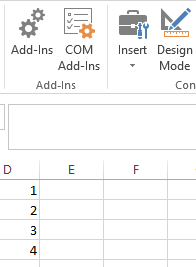## Do Until Loop

In this article we are going to learn about programming DO UNTIL loops. First we are going to learn what loops are and why they are necessary. Then we are going to learn how to use a DO UNTIL LOOP.

Note: In excel VBA they are two types of DO LOOPS: DO WHILE and DO UNTIL. Our focus will be on the DO UNTIL loop in this article.Consider the following code:

```Dim numbcount As Integer
numbcount = 1 + 2 + 3 + 4 + 5 + 6 + 7 + 8 + 9 + 10```

This code is used to add the numbers from 1 to 10. The result is then stored in the variable numbcount. Now this looks pretty easy and simple right. But now suppose instead of the first ten number you want to add numbers 1 to 1000, now are you going to write all the numbers and then add them? Of course you can but that would become tedious right. So what do we do? It’s easy actually we use the handy programming tool called “Loop”.

Loop as the names sound is something that goes on and on until you tell it to stop (this is called a “condition”). You set a starting number for your loop, an end condition, and a way to get from the starting number to the end condition. In VBA, there are four types of loop to choose from: For loops, For Each loop, Do Loops, and While loops. We will focus on the DO UNTIL loop for now.

First let’s look at the syntax of the DO UNTIL LOOP:

```Do Until [condition]
Loop```

Explanation: First you declare the DO UNTIL loop by writing “Do Until” followed by square brackets in which you write a condition. This condition will be used to end your DO UNTIL loop.

Here is an example for you to understand the syntax better:

```Do Until counter > 5
counter = counter + 1
Loop```

Explanation: This code will increase the value of a variable called count by 1 and then store the value in the same variable count. This loop will keep on going again and again until the condition statement is true meaning until the value of count is less than equal to 5.

Now let’s see how to use the Do UNTIL loop. Follow the steps below:

STEP1

Right click anywhere on the ribbon and select “Customize the Ribbon” from the drop down menu.STEP2

An option dialog box will open. Click on the DEVELOPER check box (it is under “Customize the Ribbon Main Tabs”) and press ok.STEP3

The developer tap is now visible and is present next to the view tab on the top menu bar. Click on Developer tab and select “View Code”STEP4

A new window (Visual Basic Editor) will open which would have a dialog box in the center. You will write the code in the dialog box.STEP5

Write the following line of code in the dialog box

```Sub loopexample2()
Dim counter As Integer
counter = 1
Do Until counter >= 5
Cells(counter, "D").Value = counter```

After writing the code close the window by clicking on the cross(x) icon on the upper right side of the screen. Don’t worry Excel won’t close.

Explanation: In this code we have declared a variable “counter” of data type integer and has assigned the value 1 to it. We used the DO UNTIL loop with the condition to count from 1 to 4 until count value is greater than equal to 5 and store the value in cell D.STEP6

This is the result. That’s it you have now used the DO UNTIL LOOP.```Further reading:
Basic concepts
Getting started with Excel
Cell References```
Previous articleAvoid Errors Using IFERROR-Everyone Should Know
Next articleLinking Text Box To A Specific Cell